### CODE Function Examples – Excel & Google Sheets

This tutorial demonstrates how to use the Excel CODE Function in Excel to get the numeric code representing a character.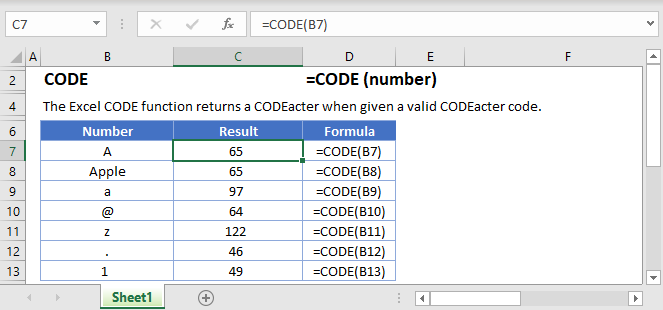## CODE Function Overview

The CODE Function Returns the numeric code for the first character in a cell containing text. This code refers to the character set on your computer.

To use the CODE Excel Worksheet Function, select a cell and type: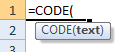(Notice how the formula inputs appear)

### CODE function Syntax and inputs:

`=CODE(text)`

text – A string of text.

AutoMacro - VBA Code Generator

## What Is the CODE Function?

A “character set” is a list of each character that your computer can display. This includes letters, numbers, special characters, and punctuation marks – as well as non-printable characters like line breaks.

Each character in the character is linked to a unique code. The Excel CODE function tells you what that code is for a given character.

### How to Use the CODE Function

Use the Excel CODE Function as shown below:

`=CODE("A")`

Excel will return 65, which is the code associated with a capital “A”.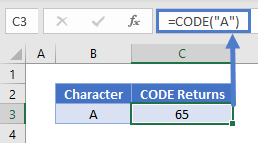As shown above, to get the code for a non-numerical character, you need to surround that character in double quotes. To get the code for a numerical character, you don’t need to use quotes: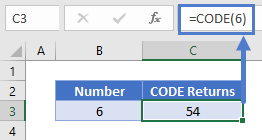### CODE Vs. CHAR

The Excel CHAR Function is the opposite of CODE – you tell CHAR a code, and it returns the associated character.

For example:

=CHAR(65)

Will return a capital “A”.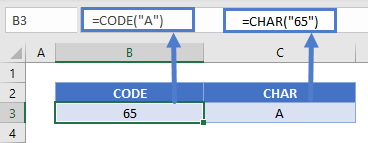A typical use of CODE is to return character codes so that they can be used in formulas with CHAR.

## CODE in Google Sheets

The CODE works exactly the same in Google Sheets as in Excel: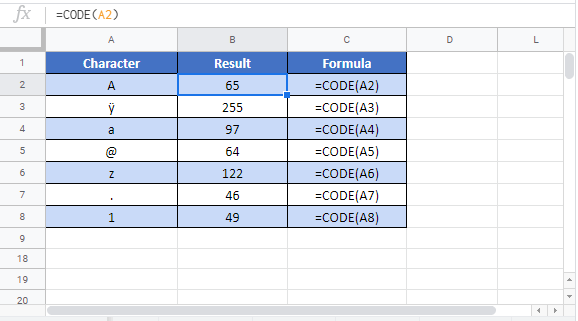## Additional Notes

The CODE Function converts a character into it’s corresponding code. The CHAR Function does the reverse. It converts a code into the corresponding character.

## Excel Practice Worksheet

Practice Excel functions and formulas with our 100% free practice worksheets!

• Automatically Graded Exercises
• Learn Excel, Inside Excel!